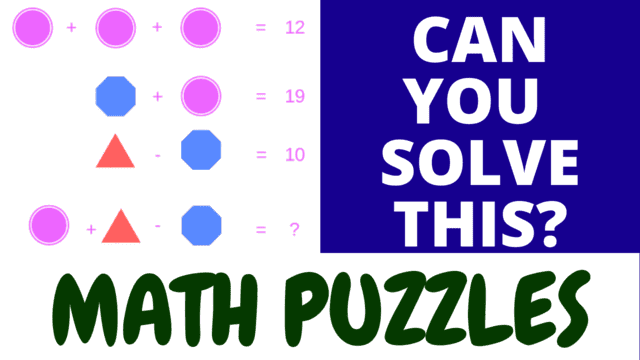Math Number Puzzle Challenge: Test Your Algebraic Skills

Put your mathematical skills to the test with our captivating Math Number Puzzle Challenge video! Solve algebraic problems, calculate variable values, and master the final equations. Can you conquer all 5 math puzzles on your first attempt? Share your solutions and enjoy the thrill of puzzle-solving!

Are you ready to embark on a mathematical journey that will push the boundaries of your skills? Our Math Number Puzzle Challenge video is designed to test your mathematical prowess with a series of engaging algebraic problems.

Within this video, you'll encounter 5 challenging algebraic problems that will put your math knowledge to the test. Your mission is two-fold: first, calculate the values of the given variables within each problem. Once you've determined the values of these variables, you'll be ready to tackle the final mathematical equation.

As you dive into these math puzzles, rely on your understanding of mathematical principles and algebraic techniques to unravel the complexities. Each problem is a unique puzzle, and your problem-solving abilities will be your greatest asset.

Challenge yourself to conquer all 5 math puzzles on your first attempt and share your solutions in the comments. Engage with fellow math enthusiasts who are equally eager to showcase their mathematical acumen.

Are you up for the challenge? Dive into the Math Number Puzzle Challenge, test your mathematical skills, and savor the satisfaction of mastering each puzzle!